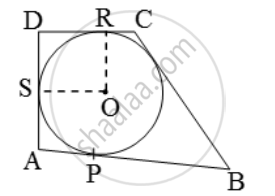# ABCD is a quadrilateral such that ∠D = 90°. A circle (O, r) touches the sides AB, BC, CD and DA at P,Q,R and If BC = 38 cm, CD = 25 cm and BP = 27 cm, find r. - Mathematics

Sum

ABCD is a quadrilateral such that ∠D = 90°. A circle (O, r) touches the sides AB, BC, CD and DA at P,Q,R and If BC = 38 cm, CD = 25 cm and BP = 27 cm, find r.

#### Solution

Since tangent to a circle is perpendicular to the radius through the point.

∴ ∠ORD = ∠OSD = 90°It is given that ∠D = 90° Also, OR = OS. Therefore, ORDS is a square.

Since tangents from an exterior point to a circle are equal in length.

∴ BP = BQ

CQ = CR and DR = DS

Now, BP = BQ

⇒ BQ = 27 [∵BP = 27 cm (Given)]

⇒ BC – CQ = 27

⇒ 38 – CQ = 27 [∵BC = 38 cm ]

⇒ CQ = 11cm

⇒ CR = 11cm [∵CR = CQ ]

⇒ CD – DR = 11

⇒ 25 – DR = 11 [∵CD = 25cm ]

⇒ DR = 14 cm

But, ORDS is a square.

Therefore, OR = DR = 14 cm

Hence, r = 14 cm

Concept: Concept of Circle - Centre, Radius, Diameter, Arc, Sector, Chord, Segment, Semicircle, Circumference, Interior and Exterior, Concentric Circles
Is there an error in this question or solution?
Share